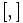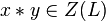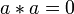# Generalized Baer correspondence

The term generalized Baer correspondence can be used for any of a number of possible generalizations of the Baer correspondence, some of which are more general than others.

The Baer correspondence (which we will call the Baer correspondence proper to distinguish it from generalizations) is a correspondence:

Uniquely 2-divisible class (at most) two Lie rings (called Baer Lie rings)$\leftrightarrow$ Uniquely 2-divisible class (at most) two groups (called Baer Lie groups)

All our generalizations share the following features:

• Each generalization has some class (at most) two Lie rings on one side and some class (at most) two groups on the other side.
• In each generalization, the Lie ring and the group have the same order, and their structure is roughly similar. In most of the generalizations discussed below, the additive group of the Lie ring is 1-isomorphic to the group, but this 1-isomorphism is not given by a unique formula as is the case with the Baer correspondence proper. Rather, there is some degree of choice which ends up not mattering.

In order to understand each generalization, we recall that for the Baer correspondence proper, the formulas are as follows:

• Group multiplication in terms of Lie ring addition:$xy := x + y + \frac{1}{2}[x,y]$ where$[ , ]$ denotes the commutator.
• Lie ring addition in terms of group multiplication:$x + y : = \frac{xy}{\sqrt{[x,y]}}$ where$[ , ]$ denotes the Lie bracket.

The elements$\frac{1}{2}[x,y]$ and$\sqrt{[x,y]}$ lie in the respective centers because center of uniquely p-divisible Lie ring is uniquely p-divisible and center of uniquely p-divisible group is uniquely p-divisible. Hence, in the latter case, it is okay to divide by this element without specifying whether the division is on the left or on the right.

Each generalization has to tackle the fundamental problem:

How do we make sense of$\frac{1}{2}[x,y]$ (respectively$\sqrt{[x,y]}$) in the absence of unique 2-divisibility? Or, what do we replace these by?

## Summary of generalizations

In general, the generalizations below are in increasing order of generality: each one generalizes all its predecessors. However, the second and third generalization (UCS-Baer and LUCS-Baer) are not more general than the first (LCS-Baer).

Name of generalized correspondence Eligible Lie rings Eligible groups Intermediate structure with extra information Key idea of generalization Rough description of extra generality offered Group(s) of smallest order covered at this level of generality but at no preceding level Order of these groups
LCS-Baer correspondence LCS-Baer Lie rings LCS-Baer Lie groups -- can do unique halving within the derived subring or derived subgroup, not necessarily in the whole group allows us to consider direct products of abelian groups (respectively, abelian Lie rings) and Baer Lie groups (respectively, Baer Lie rings). In particular, this slight generalization covers all finite nilpotent groups whose 2-Sylow subgroup is abelian and all other Sylow subgroups have class at most two. cyclic group:Z2 2
UCS-Baer correspondence UCS-Baer Lie rings UCS-Baer Lie groups -- can do unique halving within the center, not necessarily in the whole group allows us to consider central products where we fill in halves. Nothing finite. Smallest example is central product of UT(3,Z) and Q --
LUCS-Baer correspondence LUCS-Baer Lie rings LUCS-Baer Lie groups -- every element of the derived subring or subgroup has a unique half in the whole Lie ring or group (which per force must land inside the center). can halve derived subgroup/subring stuff within center, but not necessarily do "repeated halving" Nothing finite. Smallest example is central product of UT(3,Z) and Z identifying center with 2Z --
CS-Baer correspondence CS-Baer Lie rings CS-Baer Lie groups -- can do unique halving of elements of derived subring/subgroup within some intermediate subring/subgroup that is still central it allows things like central products that introduce halves in groups or Lie rings that have class two, and lack both 2-torsion and 2-divisibility Nothing finite. Smallest example is the direct product of cyclic group:Z2 and central product of UT(3,Z) and Z identifying center with 2Z --
linear halving generalization of Baer correspondence Lie ring whose bracket is the double of a Lie bracket giving nilpotency class two Group of nilpotency class two whose commutator map is the double of an alternating bihomomorphism giving class two Lie ring of nilpotency class two can find a linear half, not necessarily a unique or natural choice covers some finite and infinite non-abelian 2-groups of nilpotency class two. The smallest example is of order$2^6$, namely SmallGroup(64,57) on the group side. SmallGroup(64,57) 64
cocycle halving generalization of Baer correspondence Lie ring arising as the double of a class two Lie cring group of nilpotency class two whose commutator map is the double of a skew-symmetric cyclicity-preserving 2-cocycle class two Lie cring can find a skew-symmetric cyclicity-preserving 2-cocycle that functions as the half, not necessarily unique or natural choice covers some finite and infinite non-abelian 2-groups of nilpotency class two M16 and central product of D8 and Z4 16
cocycle skew reversal generalization of Baer correspondence Lie ring arising as the skew of a class two near-Lie cring group of nilpotency class two whose commutator map is the skew of a cyclicity-preserving 2-cocycle class two near-Lie cring can find a cyclicity-preserving 2-cocycle with the Lie bracket/commutator as skew, not necessarily skew-symmetric covers some finite and infinite non-abelian 2-groups of nilpotency class two not covered by the previous examples SmallGroup(32,2) 32

## Division unique if starting within the derived subgroup/subring

### Derived subgroup/subring is uniquely 2-divisible

Further information: LCS-Baer correspondence

This is a slight generalization of the Baer correspondence:

LCS-Baer Lie rings$\leftrightarrow$ LCS-Baer Lie groups

Here:

### Elements in the derived subgroup/subring can be uniquely divided by 2 in a slightly bigger central subgroup/subring

Further information: CS-Baer correspondence

This is a further slight generalization:

CS-Baer Lie rings$\leftrightarrow$ CS-Baer Lie groups

Here:

• A CS-Baer Lie ring is a Lie ring of nilpotency class two$L$ such that there exists a Lie subring$K$ such that$[L,L] \le K \le Z(L)$, and every element of$L$ has a unique half in$K$.
• A CS-Baer Lie group is a group of nilpotency class two$G$ such that there exists a subgroup$H$ such that$[G,G] \le H \le Z(G)$, and every element of$[G,G]$ has a unique square root within$H$.

### Other related generalizations

• The LUCS-Baer correspondence is one where every element of the derived subgroup (or subring) can be halved uniquely in the whole group, and hence uniquely within the center (since center is local powering-invariant). This is a special case of the CS-Baer correspondence but incomparable with the LCS-Baer correspondence.
• The UCS-Baer correspondence is one where unique halving is possible within the whole center, for all elements in the center. This is stronger than the LUCS-Baer correspondence but incomparable with the LCS-Baer correspondence.

## Linear halving generalization

Further information: linear halving generalization of Baer correspondence

The key idea behind this generalization is to find a new candidate for the commutator (or Lie bracket) which still gives a class two structure, and whose square (or double) is the original commutator (or Lie bracket). The correspondence is thus:

Lie rings whose brackets arise as the double of a Lie bracket giving nilpotency class two$\leftrightarrow$ Groups whose commutator arises as the square of an alternating bihomomorphism of class two

### From Lie ring to group

Suppose$L$ is a Lie ring of class at most two. Suppose we find a function:$*:L \times L \to L$

satisfying the following conditions:

•$x * y \in Z(L)$ for all$x,y \in L$.
•$x * y = 0$ whenever$x \in L$ and$y \in Z(L)$.
•$*$ is biadditive, i.e., it is$\mathbb{Z}$-bilinear. In other words,$a * (b + c) = (a * b) + (a * c)$ and$(a + b) * c = (a * c) + (b * c)$ for all$a,b,c \in L$.
•$*$ is alternating, i.e.,$a * a = 0$ for all$a \in L$. This also implies that$*$ is skew symmetric, i.e.,$a * b = -(b * a)$ for all$a,b \in L$.
•$2(x * y) = [x,y]$ for all$x,y \in L$.

Equivalently, we can state the above as:$*$ is a Lie bracket on$L$ that gives$L$ the structure of a Lie ring of class at most two, and such that$2(*) = [ , ]$.

Then, we can define the Lie group corresponding to$L$ by the multiplication$\cdot$:$x \cdot y := x + y + (x * y)$

### From group to Lie ring

Similar to the above. PLACEHOLDER FOR INFORMATION TO BE FILLED IN: [SHOW MORE]

## Cocycle halving generalization

Further information: cocycle halving generalization of Baer correspondence

### From Lie ring to group

Suppose$L$ is a Lie ring of class at most two. Suppose we find a function:$*:L \times L \to L$

satisfying the following conditions:

•$x * y \in Z(L)$ for all$x,y \in L$.
•$x * y = 0$ whenever$x \in L$ and$y \in Z(L)$.
•$*$ is a 2-cocycle for trivial group action of$L$ on itself. (Along with the previous two conditions, this would imply that it descends to a 2-cocycle for trivial group action of$L/Z(L)$ on$Z(L)$).
•$x * y = 0$ whenever$x, y$ additively generate a cyclic subgroup of$L$.
•$x * y = -(y * x)$ for all$x,y \in L$.
•$2(x * y) = [x,y]$ for all$x,y \in L$.

Then, we define the group operation$\cdot$ by:$x \cdot y := x + y + (x * y)$

## Cocycle skew reversal generalization

Further information: cocycle skew reversal generalization of Baer correspondence

### From Lie ring to group

Suppose$L$ is a Lie ring of class at most two. Suppose we find a function:$*:L \times L \to L$

satisfying the following conditions:

•$x * y \in Z(L)$ for all$x,y \in L$.
•$x * y = 0$ whenever$x \in L$ and$y \in Z(L)$.
•$*$ is a 2-cocycle for trivial group action of$L$ on itself. (Along with the previous two conditions, this would imply that it descends to a 2-cocycle for trivial group action of$L/Z(L)$ on$Z(L)$).
•$x * y = 0$ whenever$x, y$ additively generate a cyclic subgroup of$L$.
•$(x * y) -(y * x) = [x,y]$ for all$x,y \in L$.

Then, we define the group operation$\cdot$ by:$x \cdot y := x + y + (x * y)$